##### Question

In: Statistics and Probability

# Salaries for teachers in a particular elementary school district are normally distributed with a mean of...

Salaries for teachers in a particular elementary school district are normally distributed with a mean of $44,000 and a standard deviation of$6,500. We randomly survey ten teachers from that district. Find the 85th percentile for the sum of the sampled teacher's salaries to 2 decimal places.

## Solutions

##### Expert Solution

Given that, mean (μ) = $44000 and standard deviation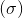=$6500

X ~ N(44000, 6500)

We want to find, the value of x such that, P(X ≤ x) = 0.85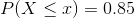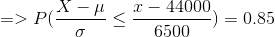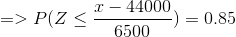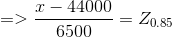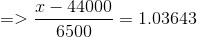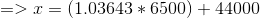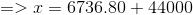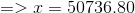##### Salaries for teachers in a particular elementary school district are normally distributed with a mean of...
Salaries for teachers in a particular elementary school district are normally distributed with a mean of $46,000 and a standard deviation of$4,900. We randomly survey ten teachers from that district. (Round your answers to the nearest dollar.) (a) Find the 90th percentile for an individual teacher's salary. (b) Find the 90th percentile for the average teacher's salary.
##### Salaries for teachers in a particular elementary school district are normally distributed with a mean of...
Salaries for teachers in a particular elementary school district are normally distributed with a mean of $46,000 and a standard deviation of$4,500. We randomly survey ten teachers from that district. (Round your answers to the nearest dollar.) A) Find the 90th percentile for an individual teacher's salary. B)Find the 90th percentile for the average teacher's salary.